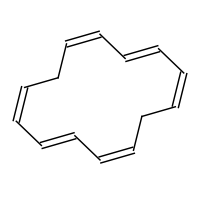# What aromatic compounds follow Hückel's rule?

Aug 7, 2014

All aromatic compounds follow Hückel's rule.

#### Explanation:

Hückel's rule states that a planar, cyclic, conjugated molecule is aromatic if it contains 4$n$ + 2 π electrons, where $n$ = 0 or any positive integer.

Compounds with $n$ = 1

The most common examples have $n$ = 1. These include

a. Benzene and its derivativesb. Six-membered heterocyclics like pyridine and pyrimidinec. Five membered heterocyclics like pyrrole, furan, and thiophened. Five-membered anions like cyclopentadienide ione. Seven-membered cations like tropylium ionCompounds with $n$ = 0cyclopropenyl cation

Compounds with $n$ = 2

These include naphthalene and azulene.Compounds with n ≥ = 3

These include the annulenes.-annulene ($n$ = 3)-annulene ($n$ = 4)